# Roses (curves)

(diff) ← Older revision | Latest revision (diff) | Newer revision → (diff)

Planar curves whose equations in polar coordinates have the form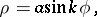whereandare constants. If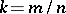is a rational number, then a rose is an algebraic curve of even order.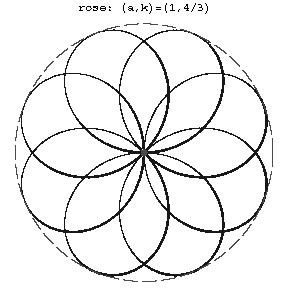Figure: r082610a

The order of a rose is equal toifandare odd, and to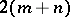if eitheroris even. The entire curve is situated inside the circle of radiusand consists of congruent parts, called petals (see Fig.). Ifis an integer, then the rose consists ofpetals forodd and ofpetals foreven. Ifand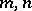are relatively prime, then the rose consists ofpetals forandodd, and of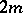petals when eitheroris even.

Whenis irrational there are infinitely many petals. Roses belong to the family of cycloidal curves (cf. Cycloidal curve). They are hypocycloids if, and epicycloids if.

Roses are also related to the family of cycloidal curves by the fact that they are pedals of epi- and hypocycloids with respect to the centre of their fixed circle.

The arc length of a rose is given by an elliptic integral of the second kind. The area of one petal is.

Roses are also called curves of Guido Grandi, who was the first to describe them in 1728.